# How to use the ISTEXT Function in Excel

In this article, we will learn about how to use the ISTEXT function in Excel.
The ISTEXT function is a logic text function. This function returns TRUE only if cell value has text or else returns False.

Syntax

=ISTEXT (value)

value : value to be checked
Note : Only one value will be given as an argument

Let’s understand this function using it in an example.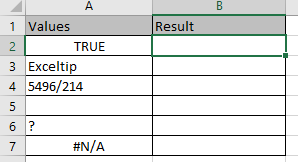Here we have a list of different values in Values column.
We need to find which of these values excel consider text.

Use the formula:

=ISTEXT(A2)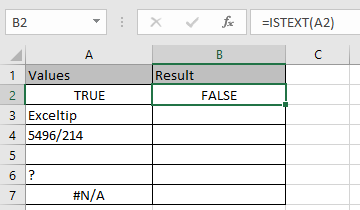ISTEXT function in excel doesn’t consider boolean logic as text.

Copy the formula in other cells using Ctrl + D shortcut key.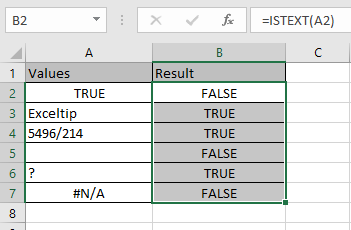As you can see from the above example that ISTEXT function returns TRUE or FALSE based on the logic test.

ISTEXT function can be used with other functions & conditional formatting.
ISTEXT function with IF function
Use the formula:

=IF(ISTEXT(A2),"Text","Not text")

ISTEXT(A2) : logic_test
"Text" : value if true
"Not text" : value if false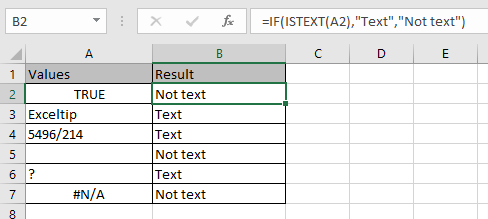As you can see the ISTEXT function with IF function.
ISTEXT function with conditional formatting

Conditional Formatting is used to highlight the data on the basis of some criteria.
Select the cells and Go to Home > Conditional formatting > New Rule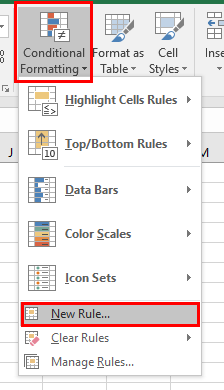Use the formula:

=ISTEXT(A2)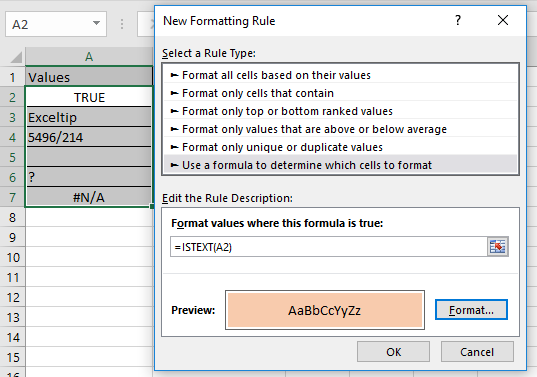Press Enter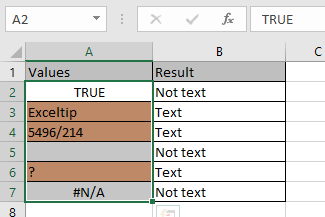As you can see the ISTEXT functions with other excel tools.

Hope you understood how to use ISTEXT function and referring cell in Excel. Explore more articles on Excel Text functions here. Please feel free to state your query or feedback for the above article.

Popular Articles:

50 Excel Shortcuts to Increase Your Productivity

How to use the VLOOKUP Function in Excel

How to use the COUNTIF in Excel 2016

How to use the SUMIF Function in Excel

Terms and Conditions of use

The applications/code on this site are distributed as is and without warranties or liability. In no event shall the owner of the copyrights, or the authors of the applications/code be liable for any loss of profit, any problems or any damage resulting from the use or evaluation of the applications/code.